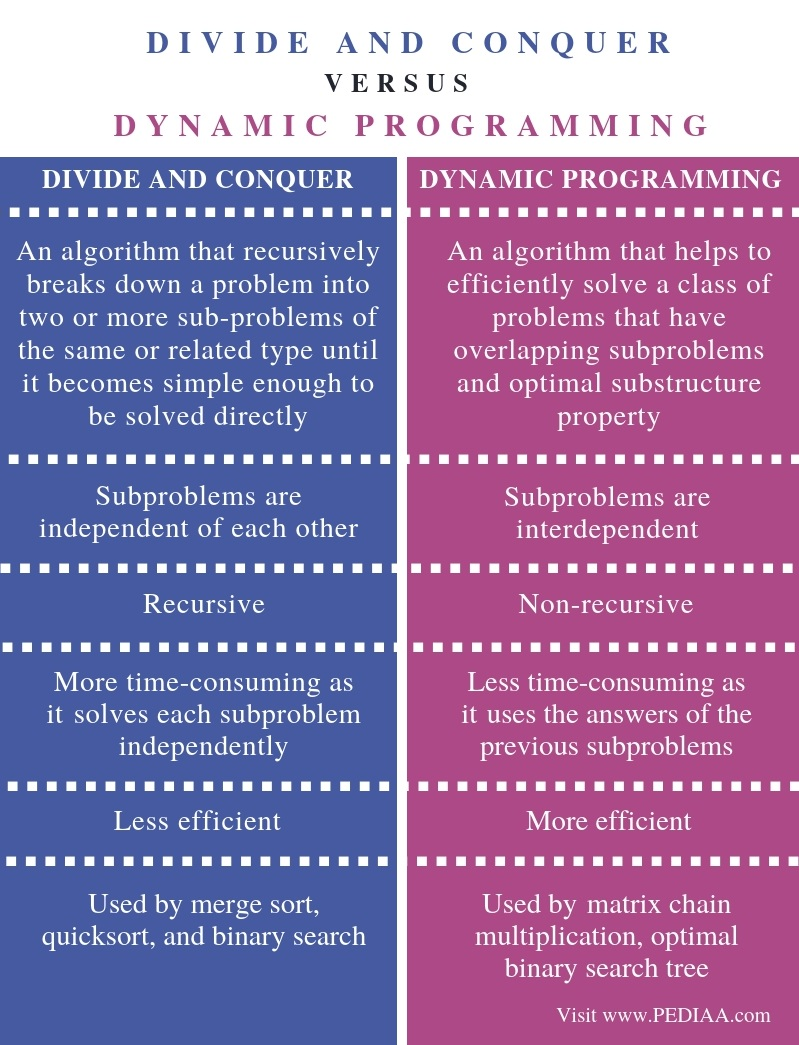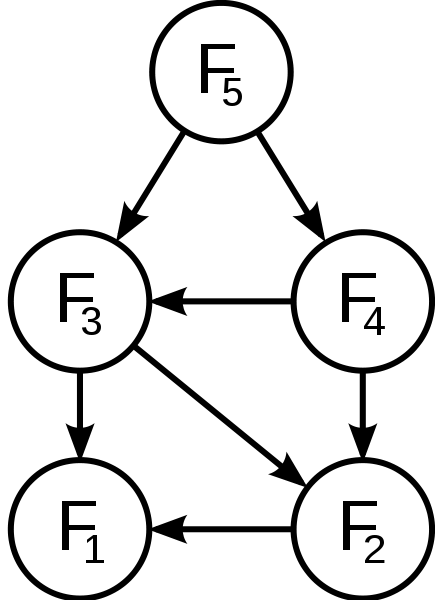# What is the Difference Between Divide and Conquer and Dynamic Programming

The main difference between divide and conquer and dynamic programming is that the divide and conquer combines the solutions of the sub-problems to obtain the solution of the main problem while dynamic programming uses the result of the sub-problems to find the optimum solution of the main problem.

Divide and conquer and dynamic programming are two algorithms or approaches to solving problems. Divide and conquer algorithm divides the problem into subproblems and combines those solutions to find the solution to the original problem. However, dynamic programming does not solve the subproblems independently. It stores the answers of subproblems to use them for similar problems.

### Key Areas Covered

1. What is Divide and Conquer
– Definition, Functionality
2. What is Dynamic Programming
– Definition, Functionality
3. What is the Difference Between Divide and Conquer and Dynamic Programming
– Comparison of Key Differences

### Key Terms

Divide and Conquer, Dynamic Programming## What is Divide and Conquer

Divide and conquer divides the main problem into small subproblems. The subproblems are divided again and again. At one point, there will be a stage where we cannot divide the subproblems further.  Then, we can solve each subproblem independently.  Finally, we can combine the solutions of all subproblems to get the solution to the main problem.There are three main steps in divide and conquer. They are as follows.

Divide (Break) – Involves splitting the main problem into a collection of subproblems

Conquer (Solve) – Involves solving each subproblem separately

Combine (Merge) – Joins the solutions of the subproblems to obtain the solution of the main problem

## What is Dynamic Programming

Dynamic programming divides the main problem into smaller subproblems, but it does not solve the subproblems independently.  It stores the results of the subproblems to use when solving similar subproblems. Storing the results of subproblems is called memorization. Before solving the current subproblem, it checks the results of the previous subproblems. Finally, it checks the results of all subproblems to find the best solution or the optimal solution.  This method is effective as it does not compute the answers again and again. Usually, dynamic programming is used for optimization.Elements of dynamic programming are as follows.

Simple subproblems – Divide the original problem into small subproblems that have a similar structure

Optimal substructure of the problem – The optimal solution to the main problem is within the optimal solution to its subproblems

Overlapping subproblems – Situations of solving the same subproblems again and again

## Difference Between Divide and Conquer and Dynamic Programming

### Definition

Divide and conquer is an algorithm that recursively breaks down a problem into two or more sub-problems of the same or related type until it becomes simple enough to be solved directly. However, dynamic programming is an algorithm that helps to efficiently solve a class of problems that have overlapping subproblems and optimal substructure property.

### Type

The main difference between divide and conquer and dynamic programming is that divide and conquer is recursive while dynamic programming is non-recursive.

### Subproblems

In divide and conquer, the subproblems are independent of each other. However, in dynamic programming, the subproblems are interdependent. Hence, this is another major difference between divide and conquer and dynamic programming.

### Time Consumption

Time consumption is another difference between divide and conquer and dynamic programming. Divide and conquer solves each subproblem independently. Therefore, it is more time-consuming. Dynamic programming, on the other hand, uses the answers of the previous subproblems. Thus, it is less time-consuming.

### Efficiency

Efficiency also makes a difference between divide and conquer and dynamic programming. Dynamic programming is more efficient than divide and conquer.

### Applications

Merge sort, quicksort, and binary search use divide and conquer while matrix chain multiplication and optimal binary search tree use dynamic programming.

### Conclusion

The main difference between divide and conquer and dynamic programming is that the divide and conquer combines the solutions of the subproblems to obtain the solution of the main problem while dynamic programming uses the result of the subproblems to find the optimum solution of the main problem.

##### Reference:

1. “DAA Divide and Conquer Introduction – Javatpoint.” Www.javatpoint.com, Available here.
2. “Dynamic Programming Introduction – Javatpoint.” Www.javatpoint.com, Available here.
3. Dynamic Programming | Steps to Design & Applications |, Education 4u, 2 Apr. 2018, Available here.
4. “Dynamic Programming”, Programiz. com, Available here.

##### Image Courtesy:

1. “Merge sort algorithm diagram” By VineetKumar at English Wikipedia – Transferred from en.wikipedia to Commons by Eric Bauman using CommonsHelper (Public Domain) via Commons Wikimedia
2. “Fibonacci dynamic programming” By en:User:Dcoatzee, traced by User:Stannered – en:Image:Fibonacci dynamic programming.png (Public Domain) via Commons Wikimedia